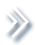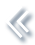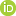####ArchivesReview Articles

## On valid descriptive inference from non-probability sample

Li-Chun Zhang

S3RI/University of Southampton, Southampton, UKL.Zhang@soton.ac.uk

Pages 103-113 | Received 05 Oct. 2018, Accepted 07 Sep. 2019, Published online: 13 Sep. 2019,
• Abstract
• References
• Citations

ABSTRACT

We examine the conditions under which descriptive inference can be based directly on the observed distribution in a non-probability sample, under both the super-population and quasi-randomisation modelling approaches. Review of existing estimation methods reveals that the traditional formulation of these conditions may be inadequate due to potential issues of under-coverage or heterogeneous mean beyond the assumed model. We formulate unifying conditions that are applicable to both types of modelling approaches. The difficulties of empirically validating the required conditions are discussed, as well as valid inference approaches using supplementary probability sampling. The key message is that probability sampling may still be necessary in some situations, in order to ensure the validity of descriptive inference, but it can be much less resource-demanding given the presence of a big non-probability sample.

## References

1. Baker, R., Brick, J. M., Bates, N. A., Battaglia, M., Couper, M. P., Dever, J. A., …Tourangeau, R. (2013). Report of the AAPOR task force on non-probability sampling (Technical Report). Deerfield, IL: American Association for Public Opinion Research. [Google Scholar]
2. Bethlehem, J. (1988). Reduction of nonresponse bias through regression estimation. Journal of Official Statistics4, 251–260. [Google Scholar]
3. Chen, J. H., & Shao, J. (2000). Nearest neighbor imputation for survey data. Journal of Official Statistics16, 113–131. [Google Scholar]
4. Deville, J.-C., & Särndal, C.-E. (1992). Calibration estimators in survey sampling. Journal of the American Statistical Association87, 376–382. doi: 10.1080/01621459.1992.10475217 [Taylor & Francis Online][Web of Science ®], [Google Scholar]
5. Elliott, M. R., & Valliant, R. (2017). Inference for nonprobability samples. Statistical Science32, 249–264. doi: 10.1214/16-STS598 [Crossref][Web of Science ®], [Google Scholar]
6. Kang, J. D. Y., & Schafer, J. L. (2007). Demystifying double robustness: A comparison of alternative strategies for estimating a population mean from incomplete data (with discussion). Statistical Science22, 523–539. doi: 10.1214/07-STS227 [Crossref][Web of Science ®], [Google Scholar]
7. Kim, J. K., & Haziza, D. (2014). Doubly robust inference with missing data in survey sampling. Statistica Sinica24, 375–394. [Web of Science ®], [Google Scholar]
8. Kim, J.-K., & Rao, J. N. K. (2018). Data integration for big data analysis in finite population inference. Talk presented at SSC2018. Montreal. [Google Scholar]
9. Kim, J.-K., & Wang, Z. (2018). Sampling techniques for big data analysis in finite population inference. Retrieved from arXiv:1801.09728v1 [Google Scholar]
10. Little, R. J. A. (1982). Models for nonresponse in sample surveys. Journal of the American Statistical Association77, 237–250. doi: 10.1080/01621459.1982.10477792 [Taylor & Francis Online][Web of Science ®], [Google Scholar]
11. Meng, X. L. (2018). Statistical paradises and paradoxes in big data (I): Law of large populations, big data paradox, and 2016 US presidential election. The Annals of Applied Statistics12, 685–726. doi: 10.1214/18-AOAS1161SF [Crossref][Web of Science ®], [Google Scholar]
12. Oh, H. L., & Scheuren, F. J. (1983). Weighting adjustments for unit non-response. In W. G. Madow, I. Olkin & D. B. Rubin (Eds.), Incomplete data in sample surveys (Vol. 2): Theory and bibliographies (pp. 143–184). New York: Academic Press. [Google Scholar]
13. Pfeffermann, D. (2017). Bayes-based non-Bayesian inference on finite populations from non-representative samples. Calcutta Statistical Association Bulletin69, 1–29. doi:10.1177/0008068317696546 [Crossref], [Google Scholar]
14. Pfeffermann, D., Krieger, A. M., & Rinott, Y. (1998). Parametric distributions of complex survey data under informative probability sampling. Statistica Sinica8, 1087–1114. [Web of Science ®], [Google Scholar]
15. Rao, J. N. K. (1966). Alternative estimators in PPS sampling for multiple characteristics. Sankhya28, 47–60. [Google Scholar]
16. Rivers, D. (2007). Sampling for web surveys. Proceedings of the survey research methods section. American Statistical Association. [Google Scholar]
17. Robins, J. M., Rotnitzky, A., & Zhao, L. P. (1994). Estimation of regression coefficient when some regressors are not always observed. Journal of the American Statistical Association89, 846–866. doi: 10.1080/01621459.1994.10476818 [Taylor & Francis Online][Web of Science ®], [Google Scholar]
18. Rosenbaum, P., & Rubin, D. (1983). The central role of the propensity score in observational studies for causal effects. Biometrika70, 41–55. doi: 10.1093/biomet/70.1.41 [Crossref][Web of Science ®], [Google Scholar]
19. Royall, R. M. (1970). On finite population sampling theory under certain linear regression models. Biometrika57, 377–387. doi: 10.1093/biomet/57.2.377 [Crossref][Web of Science ®], [Google Scholar]
20. Rubin, D. B. (1976). Inference and missing data. Biometrika63, 581–592. doi: 10.1093/biomet/63.3.581 [Crossref][Web of Science ®], [Google Scholar]
21. Smith, T. M. F. (1983). On the validity of inferences from non-random sample. Journal of the Royal Statistical Society, Series A146, 394–403. doi: 10.2307/2981454 [Crossref][Web of Science ®], [Google Scholar]
22. Tam, S.-M., & Kim, J.-K. (2018a). Big data ethics and selection-bias: An official statistician's perspective. Statistical Journal of the IAOS34, 577–588. doi:10.3233/SJI-170395 [Crossref], [Google Scholar]
23. Tam, S.-M., & Kim, J.-K. (2018b). Mining big data for finite population inference. Talk presented at BigSurv18. Barcelona. [Google Scholar]
24. Yang, S., & Kim, J.-K. (2018). Integration of survey data and big observational data for finite population inference using mass imputation. Retrieved from https://arxiv.org/abs/1807.02817v1 [Google Scholar]
25. Zhang, L.-C. (2019). Proxy expenditure weights for consumer price index: Audit sampling inference for big data statistics. Retrieved from https://arxiv.org/abs/1906.11208 [Google Scholar]